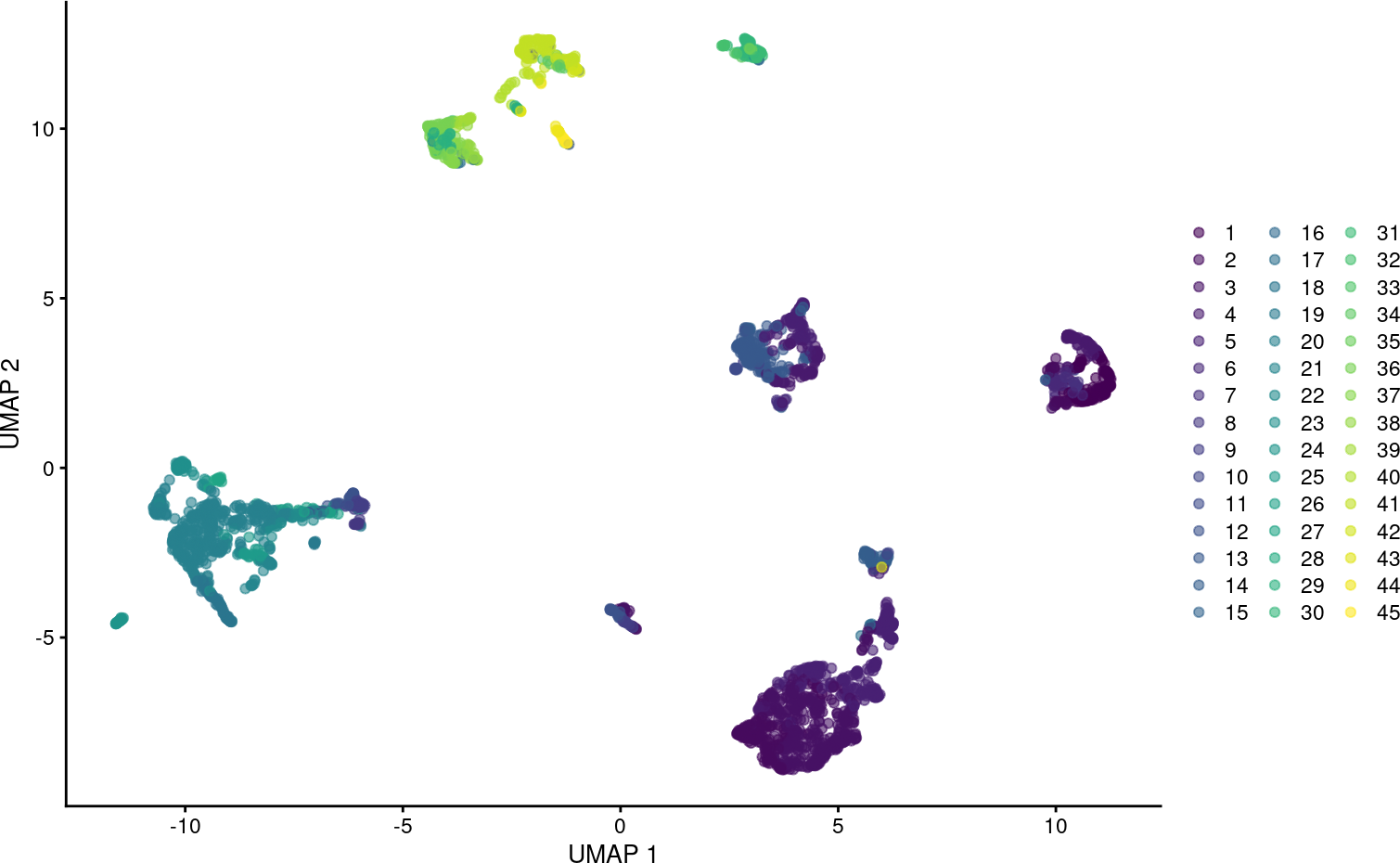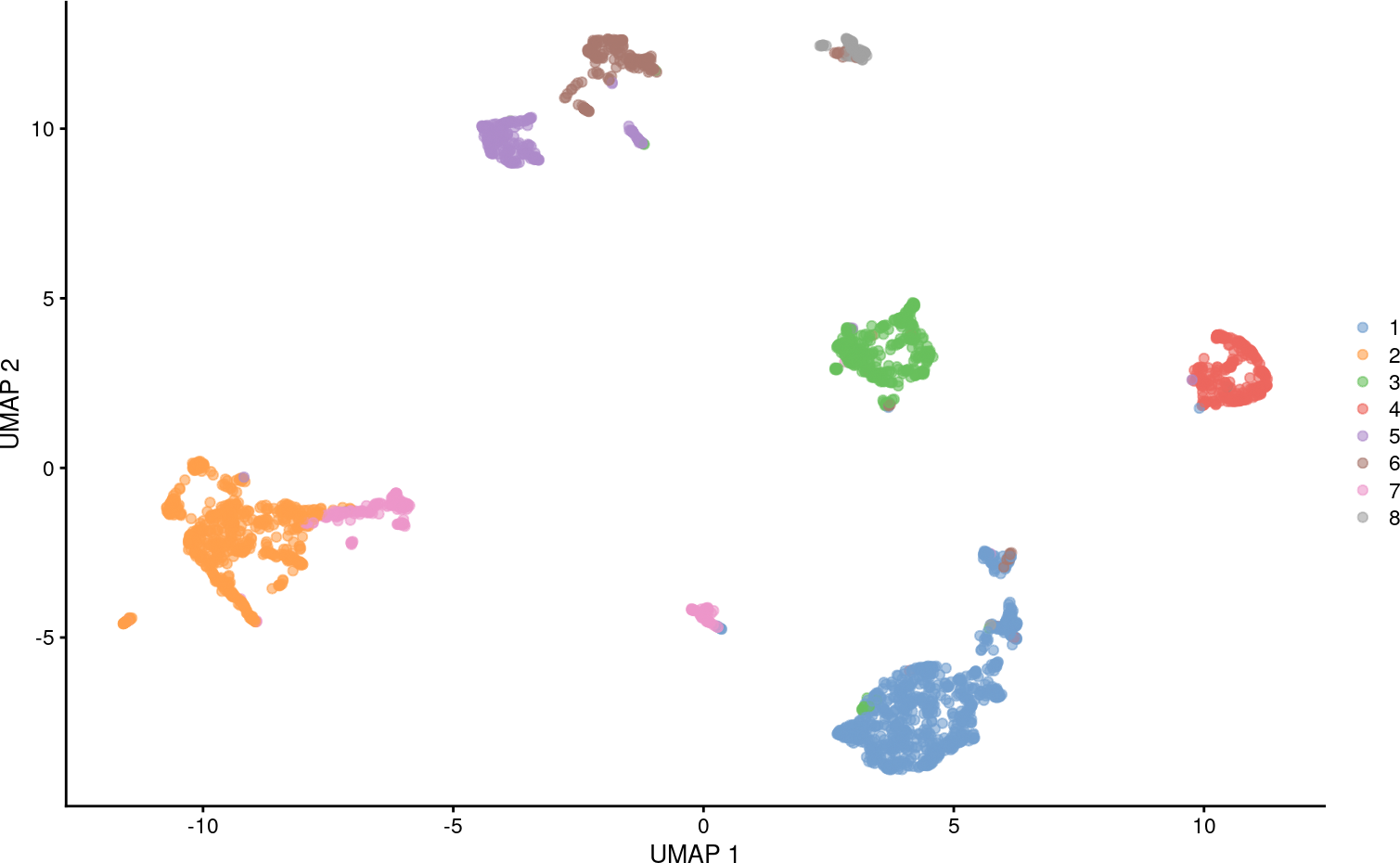# 1 Introduction

The bluster package provides a flexible and extensible framework for clustering in Bioconductor packages/workflows. At its core is the `clusterRows()` generic that controls dispatch to different clustering algorithms. We will demonstrate on some single-cell RNA sequencing data from the scRNAseq package; our aim is to cluster cells into cell populations based on their PC coordinates.

``````library(scRNAseq)
sce <- ZeiselBrainData()

# Trusting the authors' quality control, and going straight to normalization.
library(scuttle)
sce <- logNormCounts(sce)

# Feature selection based on highly variable genes.
library(scran)
dec <- modelGeneVar(sce)
hvgs <- getTopHVGs(dec, n=1000)

# Dimensionality reduction for work (PCA) and pleasure (t-SNE).
set.seed(1000)
library(scater)
sce <- runPCA(sce, ncomponents=20, subset_row=hvgs)
sce <- runUMAP(sce, dimred="PCA")

mat <- reducedDim(sce, "PCA")
dim(mat)``````
``##  3005   20``

# 2 Based on distance matrices

## 2.1 Hierarchical clustering

Our first algorithm is good old hierarchical clustering, as implemented using `hclust()` from the stats package. This automatically sets the cut height to half the dendrogram height.

``````library(bluster)
hclust.out <- clusterRows(mat, HclustParam())
plotUMAP(sce, colour_by=I(hclust.out))``````Advanced users can achieve greater control of the procedure by passing more parameters to the `HclustParam()` constructor. Here, we use Ward’s criterion for the agglomeration with a dynamic tree cut from the dynamicTreeCut package.

``````hp2 <- HclustParam(method="ward.D2", cut.dynamic=TRUE)
hp2``````
``````## class: HclustParam
## metric: [default]
## method: ward.D2
## cut.fun: cutreeDynamic
## cut.params(0):``````
``````hclust.out <- clusterRows(mat, hp2)
plotUMAP(sce, colour_by=I(hclust.out))``````## 2.2 Affinity propagation

Another option is to use affinity propagation, as implemented using the apcluster package. Here, messages are passed between observations to decide on a set of exemplars, each of which form the center of a cluster.

This is not particularly fast as it involves the calculation of a square similarity matrix between all pairs of observations. So, we’ll speed it up by taking analyzing a subset of the data:

``````set.seed(1000)
sub <- sce[,sample(ncol(sce), 200)]
ap.out <- clusterRows(reducedDim(sub), AffinityParam())
plotUMAP(sub, colour_by=I(ap.out))``````